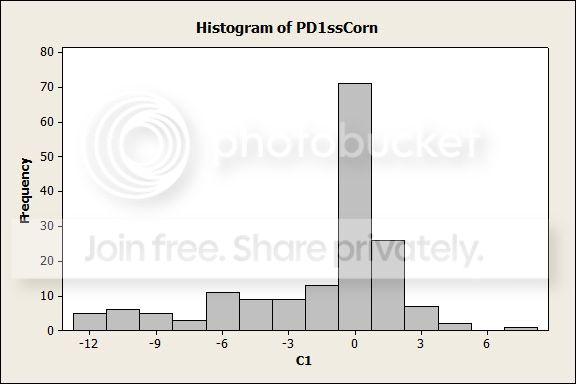# Drought index calculation

I am calculating a drought index and I have a set of values. The next step in the procedure is to standardize the values to compare across regions and time scales. The paper that establishes this particular drought index fits their dataset to the log-logistic distribution and uses the probability distribution function to standardize the values. My dataset does not follow the log-logistic distribution, or any distribution for which I can test. Is there a procedure to standardize values that do not follow a distribution?

What I am trying to do is calculate the drought index values to use in an attempt to correlate drought index with irrigation water demand.• Note that you can still standardize data that is not normally distributed - the question is whether or not the number of standard deviations from the mean (which is what you get when you standardize a variable) is meaningful for your data. What is your purpose for doing this standardization? It may also help to see a histogram or some other graphical display of the distribution of your data. – Macro Jul 27 '12 at 17:37
• Are you comparing across regions and time periods for which you yourself have collected data? If so, you may be able to make those comparisons without transforming your data. Why do you think you need to standardize your data to make comparisons? Presumably you used the same scales for temperature, etc., so what is to be gained by standardizing? If you want to compare your results to the published results, you should treat your data the way the published data was treated. Was the published data standardized? And why? – Joel W. Jul 27 '12 at 17:37
• @JoelW., I have used daily values to obtain a monthly sum. The original article for this drought index standardized the data to compare different time scales using the log-logistic probability distribution function. I am really only interested in one timescale, but since the paper standardized the values, I thought I should as well. – Jed Jul 27 '12 at 18:01
• If you are not planning to make comparisons with the published values, then perhaps you should use the analysis that makes most sense for your data set. That might not involve a transformation. (In general, I avoid transformations unless there is a compelling reason to do one.) – Joel W. Jul 27 '12 at 19:03
• I agree with Joel's comment. Further, it might be that if you estimate some sort of seasonal autoregressive model on the drought index, then the residuals might be closer to a distribution you would be familiar with. – John Jul 27 '12 at 20:44

I guess the author is referring to the Standardized Precipitation Index (SPI; McKee et al., 1993) or one derived from it (e.g., SRI (Shukla & Wood, 2008), for runoff; SSI (Hao & AghaKouchak, 2013), for soil moisture; SPEI (Vicente-Serrano et al., 2010), for precipitation and evapotranspiation; etc.). Although each of these indices was originally proposed with a specific probability distribution function (PDF), since their publication other PDFs have been used to compute them. For example, Guttman (1999) found that the Pearson type III was the best model to compute the SPI for a large data set in U. S.; meanwhile, Lana et al. (2001) found that the Poisson-gamma distribution was the best for the data analyzed in Catalonia, in Spain.

Furthermore, there are non-parametric approaches used to compute this type of drought index. For example, Farahmand & AghaKouchak (2015) proposed the use of the empirical probability estimated with the general formula for plotting positions (Hesel et al., 2020):

$$p = \frac{i - \alpha}{n - \alpha - \beta + 1}$$

where $$i$$ denotes the rank of non-zero values in the data set, from the smallest; $$n$$ is the sample size; and $$\alpha = \beta = 0.44$$.

Also, Kumar et al. (2016) used Kernel Density Estimation (KDE) with a Gaussian kernel to estimate the probability distribution of the data set.

I guess the key idea here is that you're not constrained to use a specific PDF. Rather, use the one that better fits your data, or, if each site in your analysis fits better different PDFs, use a non-parametric approach.

## References

Farahmand, A., & AghaKouchak, A. (2015). A generalized framework for deriving nonparametric standardized drought indicators. Advances in Water Resources, 76, 140–145. https://doi.org/10.1016/j.advwatres.2014.11.012

Guttman, N. B. (1999). Accepting the Standardized Precipitation Index: A calculation algorithm. Journal of the American Water Resources Association, 35(2), 311–322. https://doi.org/10.1111/j.1752-1688.1999.tb03592.x

Hao, Z., & AghaKouchak, A. (2013). Multivariate Standardized Drought Index: A parametric multi-index model. Advances in Water Resources, 57, 12–18. https://doi.org/10.1016/j.advwatres.2013.03.009

Helsel, D. R., Hirsch, R. M., Ryberg, K. R., Archfield, S. A., & Gilroy, E. J. (2020). Statistical methods in water resources. https://doi.org/10.3133/tm4a3

Kumar, R., Musuuza, J. L., van Loon, A. F., Teuling, A. J., Barthel, R., Ten Broek, J., Mai, J., Samaniego, L., & Attinger, S. (2016). Multiscale Evaluation of the Standardized Precipitation Index as a Groundwater Drought Indicator. Hydrology and Earth System Sciences, 20(3), 1117–1131. https://doi.org/10.5194/hess-20-1117-2016

Lana, X., Serra, C., and Burgueño, A.: Patterns of monthly rainfall shortage and excess in terms of the standardized precipitation index for Catalonia (Spain), Int. J. Climatol., 21, 1669–1691, 2001.

McKee, T. B., Doesken, N. J., & Kleist, J. (1993). The relationship of drought frequency and duration to time scales. Eighth Conference on Applied Climatology, 179–184. https://climate.colostate.edu/pdfs/relationshipofdroughtfrequency.pdf

Shukla, S., & Wood, A. W. (2008). Use of a Standardized Runoff Index for Characterizing Hydrologic Drought. Geophysical Research Letters, 35(2), 1–7. https://doi.org/10.1029/2007GL032487

Vicente-Serrano, S. M., Beguería, S., & López-Moreno, J. I. (2010). A multiscalar drought index sensitive to global warming: The standardized precipitation evapotranspiration index. Journal of Climate, 23(7), 1696–1718. https://doi.org/10.1175/2009JCLI2909.1